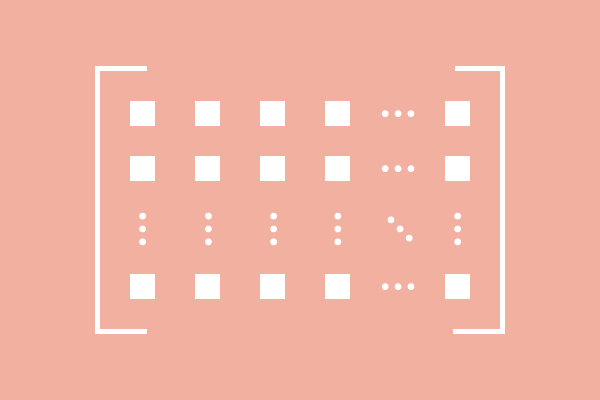# What is a Matrix?

## Definition

An object that expresses the arrangement of quantities with equal separation in horizontal and vertical directions by enclosing with brackets is called a matrix.

### Introduction

There are two fundamental rules to write the quantities in mathematics.

1. The quantities are written horizontally by separating every two quantities with a comma symbol ($,$) and some space after comma.
2. The quantities are written vertically by separating every two quantities with some space.

Now, the quantities can be arranged in the combination of both horizontal and vertical directions by the above rules. However, the commas between the quantities in horizontal lines can be ignored due to no comma symbol between the quantities in the vertical lines but some more space should be added between the quantities in horizontal direction for clear separation.

The arrangement of quantities in horizontal and vertical directions should be enclosed to form an object, and it can be done by using the brackets. Now, the arrangement of quantities in horizontal and vertical directions with equal separation and by enclosing with brackets forms an object, which is called a matrix.The quantities are usually expressed by the numbers in mathematics. So, let’s take some numbers to understand the concept of a matrix. For example, $2,$ $-1,$ $3,$ $0,$ $-4,$ $-1,$ $9,$ $7,$ $-3,$ $6,$ $-8$ and $–5$. Twelve numbers are considered in this example. Now, let’s arrange them in three rows.

$\begin{array}{rr} 2, -1, 3, 0 \\ -4, -1, 9, 7 \\ -3, 6, -8, –5 \\ \end{array}$

It seems the numbers are arranged in three rows, but it also seems the arrangement of quantities is not in proper structure because the numbers are closely appearing. However, it can be overcome by giving some space between the numbers.

$\begin{array}{rr} 2, & -1, & 3, & 0 \\ -4, & -1, & 9, & 7 \\ -3, & 6, & -8, & –5 \\ \end{array}$

Now, the arrangement of numbers in three rows is in proper structure because the quantities are properly structured in columns. So, the comma symbol is no longer required mainly due to the clear separation between the numbers.

$\begin{array}{rr} 2 & -1 & 3 & 0 \\ -4 & -1 & 9 & 7 \\ -3 & 6 & -8 & –5 \\ \end{array}$

The quantities are arranged in rows and columns, but it is essential to have something for enclosing the arrangement. So, the brackets are used to enclose the arrangement of the quantities. In mathematics, two types of brackets are used to hold the arrangement of the quantities. One is parenthesis and the second one is square brackets.

$(1).\,\,$ $\left(\begin{array}{rr} 2 & -1 & 3 & 0 \\ -4 & -1 & 9 & 7 \\ -3 & 6 & -8 & –5 \\ \end{array}\right)$

$(2).\,\,$ $\left[\begin{array}{rr} 2 & -1 & 3 & 0 \\ -4 & -1 & 9 & 7 \\ -3 & 6 & -8 & –5 \\ \end{array}\right]$

#### Examples

###### What's Next?
Latest Math Topics
Jun 26, 2023
Jun 23, 2023

###### Math Questions

The math problems with solutions to learn how to solve a problem.

Learn solutions

Practice now

###### Math Videos

The math videos tutorials with visual graphics to learn every concept.

Watch now

###### Subscribe us

Get the latest math updates from the Math Doubts by subscribing us.